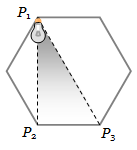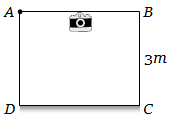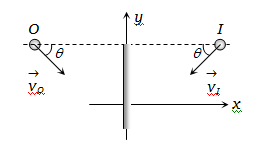The distance of the moon from earth is . The eye is most sensitive to light of wavelength 5500 Å. The minimum separation between two points on the moon that can be resolved by a 500 cm telescope will be

(a) 51 m                         (b) 60 m

(c) 70 m                          (d) All the above

Concept Questions :-

Resolution of Optical devices
High Yielding Test Series + Question Bank - NEET 2020

Difficulty Level:

A light source is located at ${P}_{1}$ as shown in the figure. All sides of the polygon are equal. The intensity of illumination at ${{P}_{2}}_{}$is ${I}_{0}$ . What will be the intensity of illumination at ${P}_{3}$ ?(a) $\frac{3}{4}{I}_{0}$                                    (b) $\frac{{I}_{0}}{8}$

(c) $\frac{3}{8}{I}_{0}$                                        (d) $\frac{\sqrt{3}}{8}{I}_{0}$

Concept Questions :-

Reflection at spherical surface
High Yielding Test Series + Question Bank - NEET 2020

Difficulty Level:

A point object is moving on the principal axis of a concave mirror of focal length 24 cm towards the mirror. When it is at a distance of 60 cm from the mirror, its velocity is 9cm/sec. What is the velocity of the image at that instant

(a) 5cm/sec towards the mirror

(b) 4cm/sec towards the mirror

(c) 4cm/sec away from the mirror

(d) 9cm/sec away from the mirror

Concept Questions :-

Reflection at spherical surface
High Yielding Test Series + Question Bank - NEET 2020

Difficulty Level:

A concave mirror is placed on a horizontal table with its axis directed vertically upwards. Let O be the pole of the mirror and C is its centre of curvature. A point object is placed at C. It has a real image, also located at C. If the mirror is now filled with water, the image will be :

(a) Real, and will remain at C

(b) Real, and located at a point between C and $\infty$

(c) Virtual and located at a point between C and O

(d) Real, and located at a point between C and O

Concept Questions :-

Reflection at spherical surface
High Yielding Test Series + Question Bank - NEET 2020

Difficulty Level:

We wish to see inside an atom. Assuming the atom to have a diameter of 100 pm, this means that one must be able to resolved a width of say 10 p.m. If an electron microscope is used, the minimum electron energy required is about

(a) 1.5 KeV                          (b) 15 KeV

(c) 150 KeV                          (d) 1.5 KeV

Concept Questions :-

Resolution of Optical devices
High Yielding Test Series + Question Bank - NEET 2020

Difficulty Level:

A telescope has an objective lens of 10 cm diameter and is situated at a distance of one kilometre from two objects. The minimum distance between these two objects, which can be resolved by the telescope, when the mean wavelength of light is 5000 Å, is of the order of

(a) 0.5 m                        (b) 5 m

(c) 5 mm                        (d) 5 cm

Concept Questions :-

Resolution of Optical devices
High Yielding Test Series + Question Bank - NEET 2020

Difficulty Level:

Two point white dots are 1mm apart on a black paper. They are viewed by eye of pupil diameter 3 mm. Approximately, what is the maximum distance at which dots can be resolved by the eye ? [Take wavelength of light = 500 nm]

(a) 6 m                           (b) 3 m

(c) 5 m                            (d) 1 m

Concept Questions :-

Human eye
High Yielding Test Series + Question Bank - NEET 2020

Difficulty Level:

A room (cubical) is made of mirrors. An insect is moving along the diagonal on the floor such that the velocity of image of insect on two adjacent wall mirrors is . The velocity of image of insect in ceiling mirror is

(a) 10 $cm{s}^{-1}$                            (b) 20 $cm{s}^{-1}$

(c) $\frac{10}{\sqrt{2}}cm{s}^{-1}$                          (d) $10\sqrt{2}cm{s}^{-1}$

Concept Questions :-

Reflection at plane surface
High Yielding Test Series + Question Bank - NEET 2020

Difficulty Level:

Figure shows a cubical room ABCD with the wall CD as a plane mirror. Each side of the room is 3m. We place a camera at the midpoint of the wall AB. At what distance should the camera be focussed to photograph an object placed at A(a) 1.5 m                                     (b) 3 m

(c) 6 m                                         (d) More than 6 m

Concept Questions :-

Reflection at plane surface
High Yielding Test Series + Question Bank - NEET 2020

Difficulty Level:

If an object moves towards a plane mirror with a speed v at an angle $\theta$ to the perpendicular to the plane of the mirror, find the relative velocity between the object and the image(a) v                                            (b) 2v

(c) 2v cos $\theta$                                    (d) 2v sin $\theta$

Concept Questions :-

Reflection at plane surface